# For the following exercises, use Kepler's Law, which states that the square of the time, T...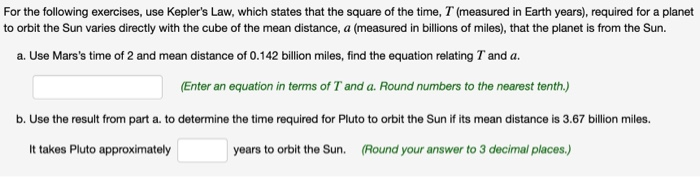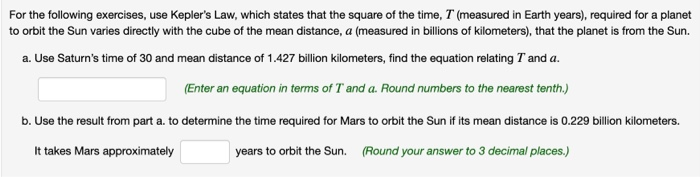For the following exercises, use Kepler's Law, which states that the square of the time, T (measured in Earth years), required for a planet to orbit the Sun varies directly with the cube of the mean distance, a (measured in billions of miles), that the planet is from the Sun. a. Use Mars's time of 2 and mean distance of 0.142 billion miles, find the equation relating T and a. (Enter an equation in terms of T and a. Round numbers to the nearest tenth.) b. Use the result from part a. to determine the time required for Pluto to orbit the Sun if its mean distance is 3.67 billion miles. It takes Pluto approximately years to orbit the Sun. (Round your answer to 3 decimal places.)
For the following exercises, use Kepler's Law, which states that the square of the time, T (measured in Earth years), required for a planet to orbit the Sun varies directly with the cube of the mean distance, a (measured in billions of kilometers), that the planet is from the Sun. a. Use Saturn's time of 30 and mean distance of 1.427 billion kilometers, find the equation relating T and a. (Enter an equation in terms of T and a. Round numbers to the nearest tenth.) b. Use the result from part a. to determine the time required for Mars to orbit the Sun if its mean distance is 0.229 billion kilometers. It takes Mars approximately years to orbit the Sun. (Round your answer to 3 decimal places.)

Data provided in the question:

According to Kepler's Law, the square of the time "T" (measured in Earth years) required for a planet to orbit the sun varies directly with the cube of the mean distance "a" (measure in billions of miles) that the planet is from the sun, i.e.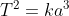where "k" is the constant.

a.

Time required by planet Mars to orbit the sun, Tm = 2

Mean distance of planet Mars from the sun, am = 0.142 billion miles.

On substituting the above values in the Kepler's Law equation, we get -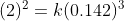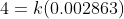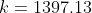Therefore, we can write the equation for Kepler's Law as -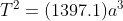b.

Mean distance of planet Pluto from the sun, ap = 3.67 billion miles.

Time required by planet Pluto to orbit the sun, Tp = ?

We can use the equation of the Kepler's Law for calculating the time required by planet Pluto to orbit the sun as -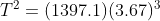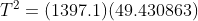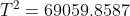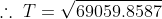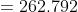Thus, the time required by planet Pluto to orbit the sun is 262.792 Earth years.

#### Earn Coin

Coins can be redeemed for fabulous gifts.

Similar Homework Help Questions
• ### Solving Direct Variation Problems For the following exercises, use Kepler's Law, which states that the square...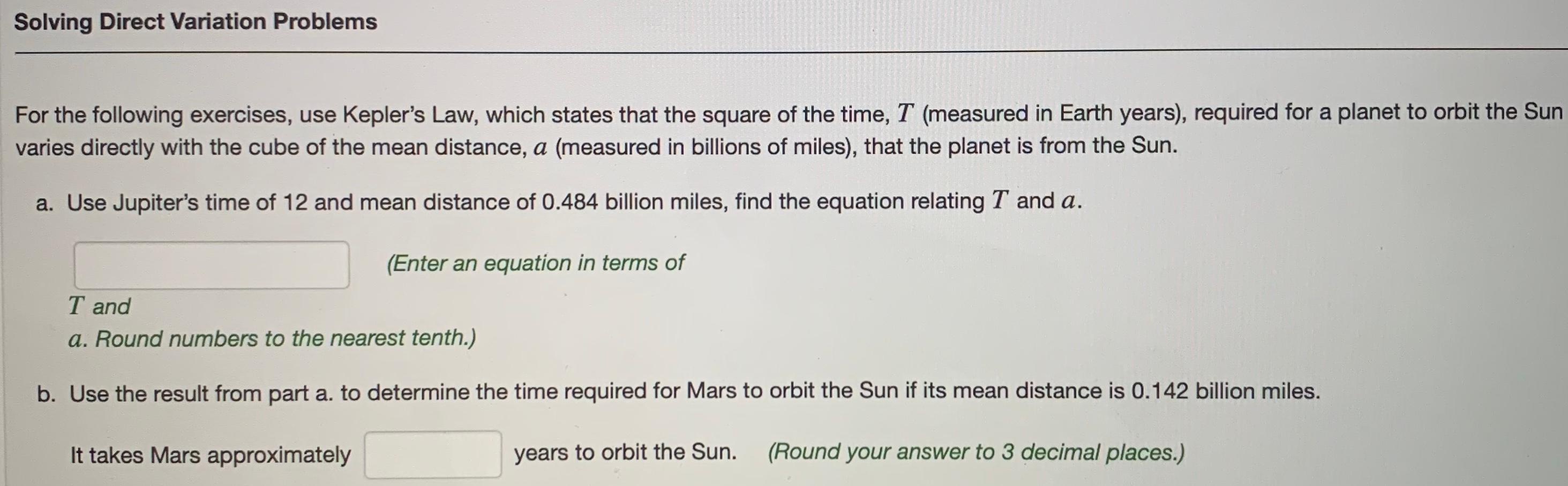Solving Direct Variation Problems For the following exercises, use Kepler's Law, which states that the square of the time, T (measured in Earth years), required for a planet to orbit the Sun varies directly with the cube of the mean distance, a (measured in billions of miles), that the planet is from the Sun. a. Use Jupiter's time of 12 and mean distance of 0.484 billion miles, find the equation relating T and a. (Enter an equation in terms of...

• ### Solving Direct Variation Problems For the following exercises, use Kepler's Law, which states that the square...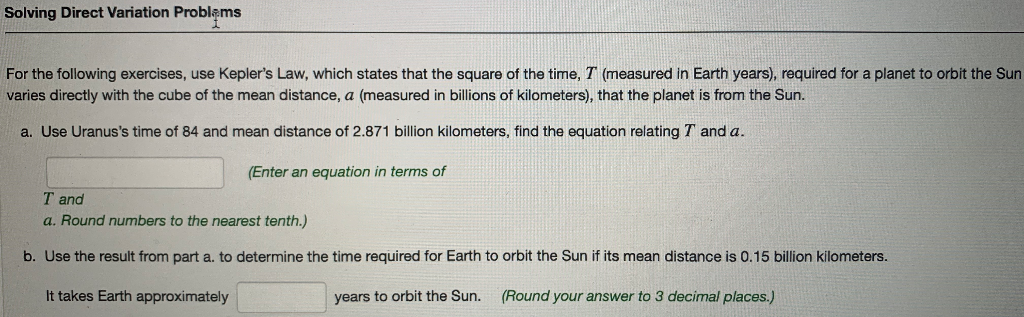Solving Direct Variation Problems For the following exercises, use Kepler's Law, which states that the square of the time, T (measured in Earth years), required for a planet to orbit the Sun varies directly with the cube of the mean distance, a (measured in billions of kilometers), that the planet is from the Sun. a. Use Uranus's time of 84 and mean distance of 2.871 billion kilometers, find the equation relating T and a. (Enter an equation in terms of...

• ### please answer and show work for all parts of equation Kepler's Law, which states that the...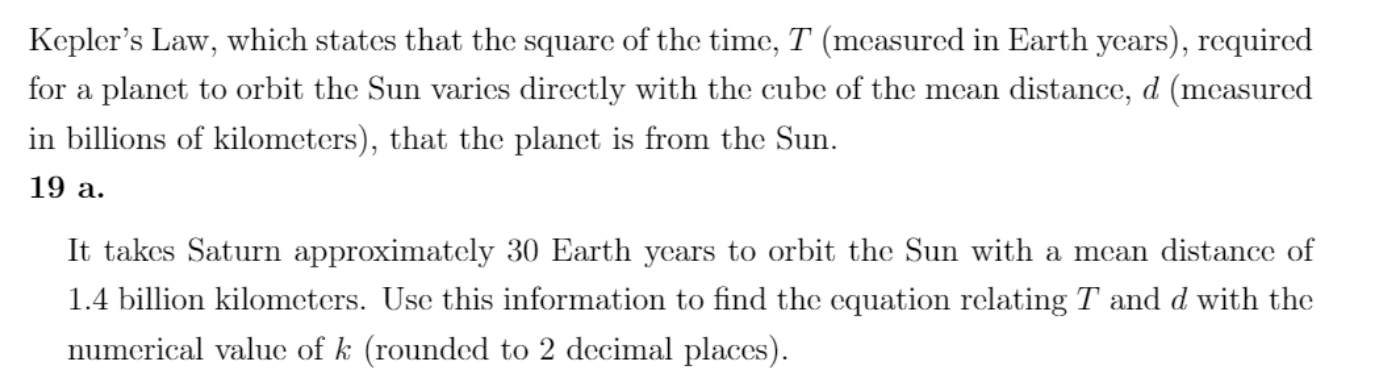please answer and show work for all parts of equation Kepler's Law, which states that the square of the time, T (measured in Earth years), required for a planet to orbit the Sun varies directly with the cube of the mean distance, d (measured in billions of kilometers), that the planet is from the Sun. 19 a. It takes Saturn approximately 30 Earth years to orbit the Sun with a mean distance of 1.4 billion kilometers. Use this information to...

• ### Pluto's distance P(t)(in billions of kilometers) from the sun as a function of time t (in...

Pluto's distance P(t)(in billions of kilometers) from the sun as a function of time t (in years) can be modeled by a sinusoidal expression of the form a.sin(b.t)d At year t=0, Pluto is at its average distance from the sun, which is 6.9 billion kilometers. In 66 years, it is at its closest point to the sun, which is 4.4 billion kilometers away. Find P(t)

• ### 1 (10 pts). In 1601 the German astronomer Johannes Kepler became director of the Prague Observatory....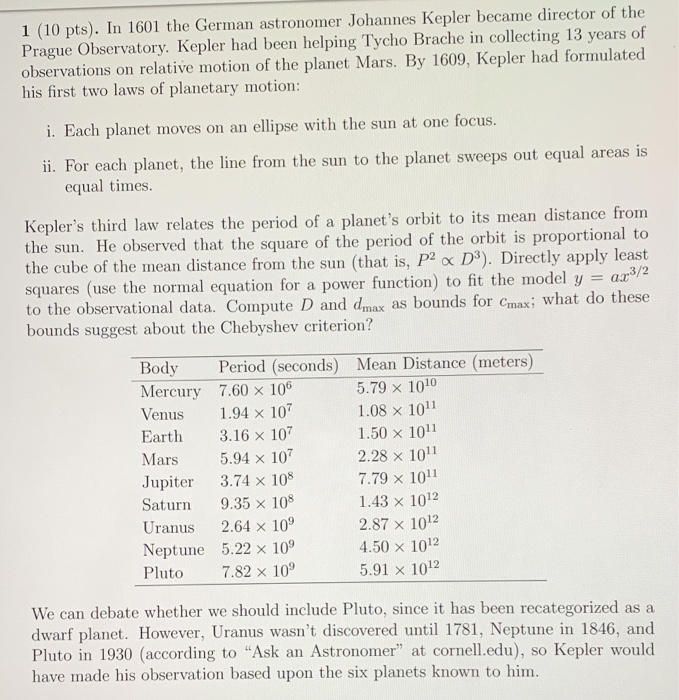1 (10 pts). In 1601 the German astronomer Johannes Kepler became director of the Prague Observatory. Kepler had been helping Tycho Brache in collecting 13 years of observations on relative motion of the planet Mars. By 1609, Kepler had formulated his first two laws of planetary motion: i. Each planet moves on an ellipse with the sun at one focus. ii. For each planet, the line from the sun to the planet sweeps out equal areas is equal times. Kepler's...

• ### The period of revolution of a planet around the sun is the time it takes for the planet to complete one orbit of the sun

The period of revolution of a planet around the sun is the time it takes for the planet to complete one orbit of the sun. The period, P years, is give by Kepler's third law P^2=D^3, where D is the average distance from the sun in astronomical units (AU). One AU is the average distance f the Earth fromt he sun. Use the average distance formt he sun to find the period of revolution for Jupiter; 5.20 AU to the...

• ### Newton's version of Kepler's Law Force Example Use what we know about the earth's orbit to estimate the...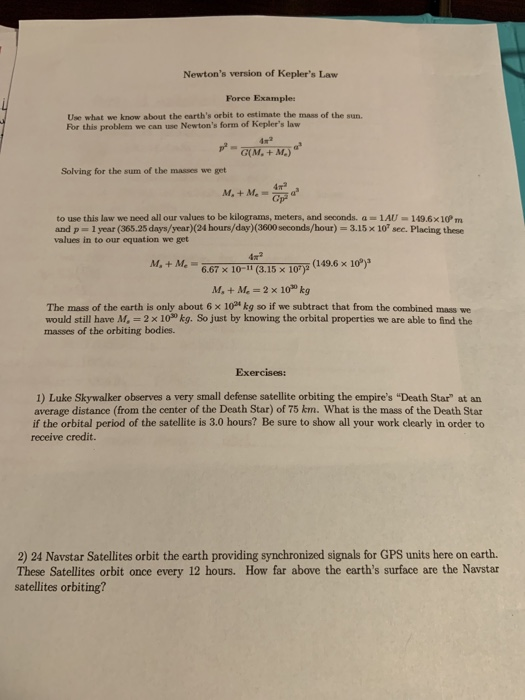Newton's version of Kepler's Law Force Example Use what we know about the earth's orbit to estimate the mass of the sun. For this problem we can use Newton's form of Kepler's law Solving for the sum of the masses we get to use this law we need all our values to be kilograms, meters, and seconds. a 1AU-149.6x10P m and p- 1 year (365.25 days/year)(24 hours/day)(3600 seconds/hour)-3.15x 10" sec. Placing these values in to our equation we get M+...

• ### С https://s3.us-east-1.amazonaws.com/blackboard.learn.xythos.prod/5a3199fc4282a/8892687?res ontent-disposition-inline%3B%20filename%2A%3DUTF-8%27%.27HW3%25285%2 Q Magnifier + 100% views repor ing I ne weights ol p irchased...С https://s3.us-east-1.amazonaws.com/blackboard.learn.xythos.prod/5a3199fc4282a/8892687?res ontent-disposition-inline%3B%20filename%2A%3DUTF-8%27%.27HW3%25285%2 Q Magnifier + 100% views repor ing I ne weights ol p irchased inere handse. Scales are allowed to opera e as long as he percent error Úr their reported weights is within 0.5% of the actual weight measured. If you wanted to purchase 15.0 pounds of flour, what range of weights is the scale allowed to report in order to be compliant with local inspection agency regulations. 3) Kepler's 3rd Law of planetary motion states that...

• ### Multivariable Calculus help with the magnitude of angular momentum: My questions is exercise 4 but I have attached exercise 1 and other notes that I was provided 4 Exercise 4. In any mechanics proble...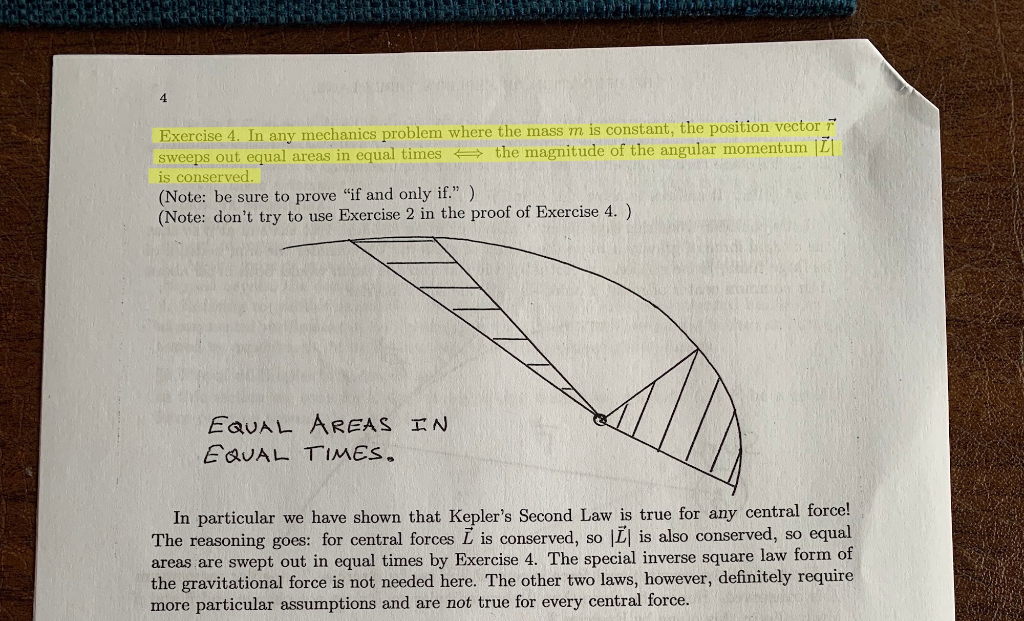Multivariable Calculus help with the magnitude of angular momentum: My questions is exercise 4 but I have attached exercise 1 and other notes that I was provided 4 Exercise 4. In any mechanics problem where the mass m is constant, the position vector F sweeps out equal areas in equal times the magnitude of the angular momentum ILI is conserved (Note: be sure to prove "if and only if") (Note: don't try to use Exercise 2 in the proof of...

• ### could you please solve a and b? Chapier 2i. Note: you needn't derive Kepler's laws-but do...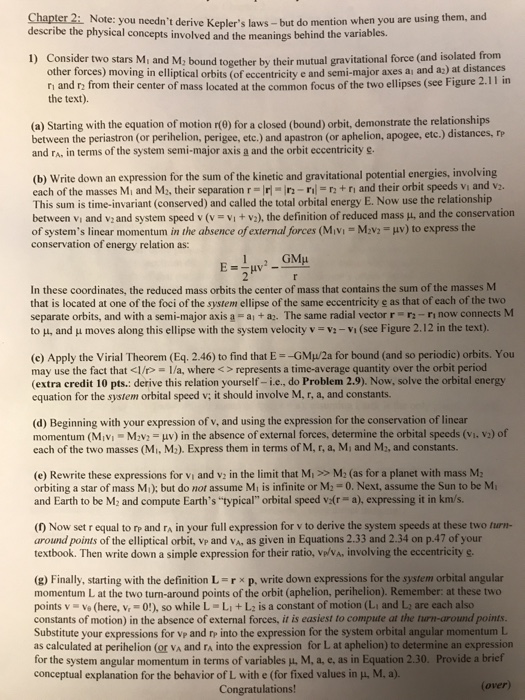could you please solve a and b? Chapier 2i. Note: you needn't derive Kepler's laws-but do mention when you are using them, an describe the physical concepts involved and the meanings behind the variables. u) Consider two stars Mi and M; bound together by their mutual gravitational force (and isolated from other forces) moving in elliptical orbits (of eccentricity e and semi-major axes ai and az) at distances 11 in n and r from their center of mass located at...

Free Homework App